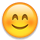# How much power does inverter draw?

I am a bit confused on how much power things draw from my (very small) 12 volt system. I have a 100 Ah battery and a 50 watt solar panel. I use a 1500 watt inverter. I know the inverter draws .5 amp when it is on, but idle.

What I don't know is: how much power does the inverter use (in addition to what the lamp draws) when it is making a/c--say, for a 20 watt lamp?

also:

If I run a 120 watt a/c appliance for an hour, does it drain 1 amp hour from my battery (since it's 120 watt a/c), or does it drain 10 amp hours (since the inverter is using 12 volt power?

Re: How much power does inverter draw?
I am a bit confused on how much power things draw from my (very small) 12 volt system. I have a 100 Ah battery and a 50 watt solar panel. I use a 1500 watt inverter. I know the inverter draws .5 amp when it is on, but idle.
• 12 volts * 0.5 amps = 6 watt draw
What I don't know is: how much power does the inverter use (in addition to what the lamp draws) when it is making a/c--say, for a 20 watt lamp?
• Power = 6 watts + (20 watt load / 0.85 inverter efficiency) = 29.6 watts total
If I run a 120 watt a/c appliance for an hour, does it drain 1 amp hour from my battery (since it's 120 watt a/c), or does it drain 10 amp hours (since the inverter is using 12 volt power?
• Power = Volts * Amps
• 120 Watts = 120 volts * 1 amp
• 120 watts = 12 volts * 10 amp [fixed typo--thank you greenthumb]
Using the above example:
• Power-inverter-input = 6 watts + (120 watts / 0.85) = 147 watts DC load
• I = P/V = 147 Watts / 12 volts = 12.25 amps
-Bill
Near San Francisco California: 3.5kWatt Grid Tied Solar power system+small backup genset
• Re: How much power does inverter draw?

Thank you so much for your clear, concise explanation!!:D:D

(You might want to correct a typo in your formula, which reads:
120 watts = 12 volts * 1 amp)
Re: How much power does inverter draw?

Thank you... Typo Fixed.

By the way, the above are all approximations... Inverters are typically less efficient near 100% loading, current input requirements change vs battery bank voltage (10.5-15.5 volts), etc.

But it does show way a large inverter (high idling losses) with small loads is not a good thing for a small system.

-Bill
Near San Francisco California: 3.5kWatt Grid Tied Solar power system+small backup genset
• Re: How much power does inverter draw?

It's a very dynamic system . . . hard to pin down exact numbers, but approximations help a lot!!
But it does show why a large inverter (high idling losses) with small loads is not a good thing for a small system.

Yeah--it didn't take long to figure that out!!
• Solar Expert Posts: 1,477 ✭✭✭✭
Re: How much power does inverter draw?

There are basically two pieces to inverter overhead.

The first one you already mentioned, in your 0.5 amps, just for the electronics control and switching of the gate control of the output MOSFET's.

A pure sinewave inverter generally has higher idle consumption then a modified sinewave because the electronic control circuitry is a bit more complicated and the output MOSFET's transistors and being chopped at a much higher frequency then a modifed sinewave inverter. A sinewave inverter has an output filter to remove the high frequency pulse width modulation so there is a clean sinewave output. This filter draws some output current through the filter capacitor whether there is an output load on the inverter or not.

The larger, the higher the wattage rating the inverter, the more idle power it generally consumes. Overkill on inverter size can hurt you in low power use cases.

The second part is dynamic, being a function of the load on the inverter.

Most of this dynamic loss is in resistive losses in the series equivalent resistance of the output MOSFET transistors, wire resistance in transformer, and interconnects. These losses will be in direct proportion to the output load current.

There is also dynamic loss in transformer core material. The transformer core losses usually don't become signficant until you are operating above 80% of the inverter rated power level.

You might think of dynamic losses as voltage drop (and associated power loss) in a long extension cord. Up to the point where transformer core losses start to become significant, the long extension cord analogy is very accurate.

At the risk of giving you more info then you want to know, power factor comes into play on inverter dynamic losses.

Let's say you have a Kill-A-Watt meter hooked up you your refrig and it tells you your refrig draws 160 watts with a power factor of 0.8. The V*A of the refrig is 200 watts. The inverter dynamic losses will be as if you are running a 200 watt load. Do not confuse this as 200 watts is translated back to battery, only that the inverter dynamic loss will be higher then if it was running a 160 watt, PF=1 load.

A bi-directional inverter, like a Xantrex XW series, or Outback FX series, react a little different to poor PF then a non bi-directional inverter like a true sinewave Power-To-Go model. The non bi-directional inverter will have a bit higher dynamic losses due to poor power factor. They also can be damaged if power factor is too poor.
• Re: How much power does inverter draw?

I think I get the gist of what you're saying, but you use a lot of terminology that's over my head . . .